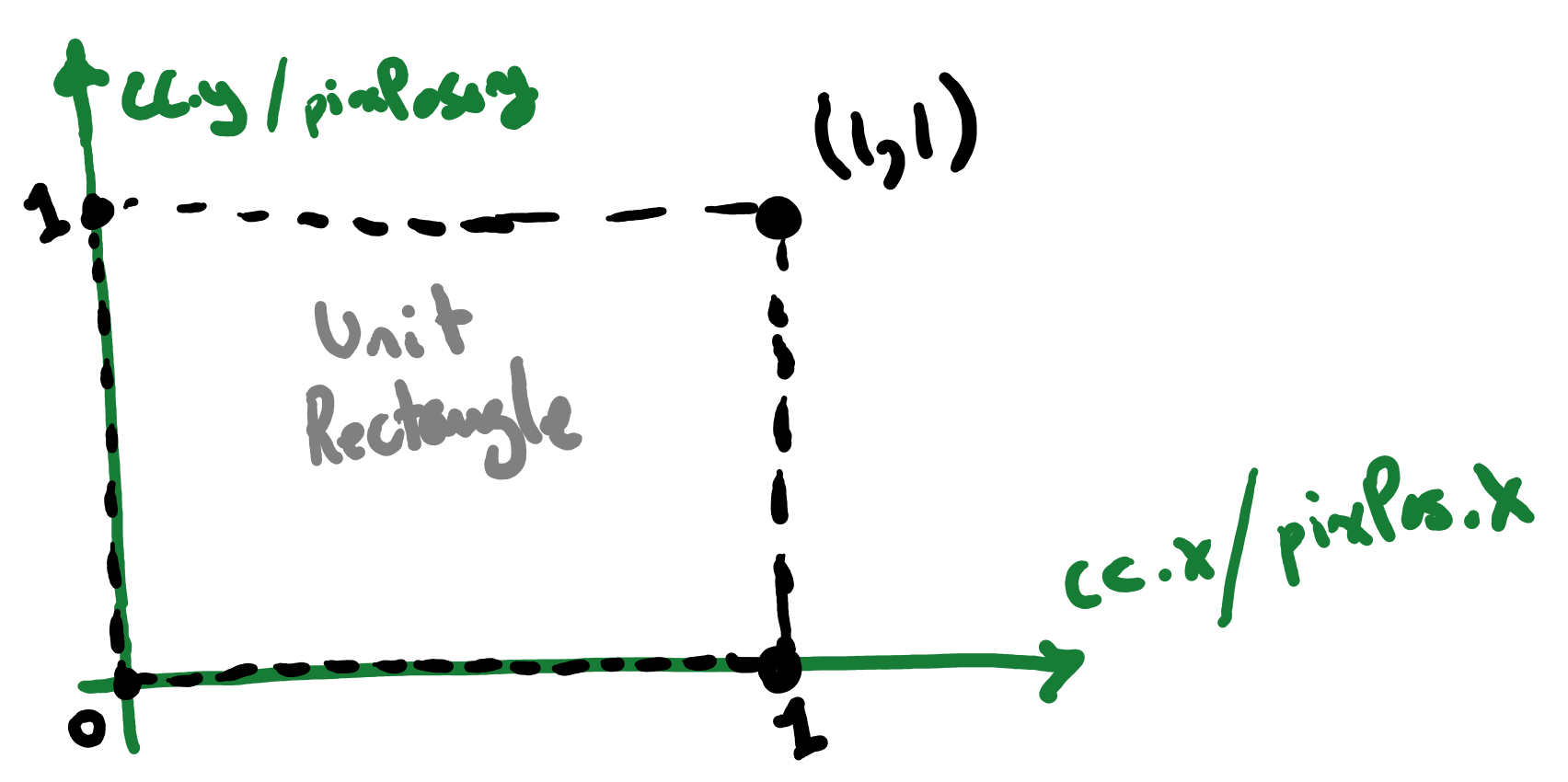Abubu.js
The WebGL Computational Library## Pixel positions

A fundamental necessity in numerical calculations is to know the position of each pixel in the fragment shader. One of the capabilities of the vertex shader is to interpolate values based on vertex data over the geometry. We can use the position of the vertices and use the interpolate the values to find the position of each pixel. The output of the vertex shader for such values will be the input of the fragment shader. he output variables are marked with out keyword and the input variables are marked with in.

We modify the vertex shader from the previous example to:

#version 300 es
precision highp float ; // high percision for float variables
precision highp int ;   // high percision for integer variables

in vec4 position;   // position of vertices as input of the shader

out vec2 pixPos ;   // pixel positions (out to fragment shader)
out vec2 cc    ;    // pixel positions (out to fragment shader)
// Main body of the vertex shader
void main() {
pixPos = position.xy ;  // interpolate based on xy values
cc     = position.xy ;  // interpolate based on xy values
gl_Position = vec4(
position.x*2.-1.,   /* x-coordinate */
position.y*2.-1.,   /* y-coordinate */
position.z,         /* z-coordinate */
1.0);
}


The above vertex shader, will calculate pixPos and cc variables by interpolating the $$x$$- and $$y$$-coordinates of the position of the vertices.Now, we can bring pixPos and cc into the fragment shader to color the unit rectangle based on the $$x$$ coordinate of each pixel.

See our shader:

#version 300 es
precision highp float ;
precision highp int ;

out vec4 outcolor ; /*  output of the shader
pixel color         */
in vec2 pixPos ;    /* input from vertex shader */
in vec2 cc ;        /* input from vertex shader */

// Main body of the shader
void main() {
/* setting r,g,b,a values of the output color as an
opaque red */
outcolor = vec4(pixPos.x,0.,0.,1.) ;
return ;
}


As you can see in the above shader, the red channel is calculated based on the $$x$$ coordinate of the pixPos (pixel position).

The result of running the program should look like this:Try to change the green or blue channels based on the $$y$$ position of the pixels. Try also to use cc instead of pixPos.

The pixel position program

Download the source code for all tutorials